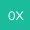Model: GPT-4```bashCopy code```pip install torch
``````

```pythonCopy code```import torch
import torch.nn as nn
import torch.optim as optim

# 定义一个简单的神经网络
def __init__(self):
self.layer = nn.Linear(2, 1)

def forward(self, x):
return self.layer(x)

# 训练数据
inputs = torch.tensor([[1.0, 1.0]])
outputs = torch.tensor([[2.0]])

# 实例化神经网络，定义损失函数和优化器
criterion = nn.MSELoss()
optimizer = optim.SGD(model.parameters(), lr=0.01)

# 训练神经网络
for epoch in range(1000):
predictions = model(inputs)
loss = criterion(predictions, outputs)
loss.backward()
optimizer.step()

if epoch % 100 == 0:
print(f'Epoch: {epoch}, Loss: {loss.item()}')

# 测试神经网络
test_input = torch.tensor([[1.0, 1.0]])
result = model(test_input)
print(f"1 + 1 = {result.item()}")
``````